Latest Banking jobs   »   Quantitative Aptitude Quiz For SIDBI Grade...

# Quantitative Aptitude Quiz For SIDBI Grade A Prelims 2023- 5th January

Q1. A and B started the work alternatively starting with A. On last day, A completed the work by doing 12.5% of the whole work. Which of the following can be the possible value of time taken by B alone to do that work if A alone can do the whole work in 6 days.
(a) 15 days
(b) 8 days
(c) 10 days
(d) 6 days
(e) 12 days

Q2. Ratio of age of Ravi to Vicky, 4 years ago was 5: 6, while ratio of present age of Rocky to that of Vicky is 5: 4. If 2 years later sum of age of Ravi and Rocky will be 63 years, then find the difference between present age of Ravi and Vicky?
(a) 4 years
(b) 2 years
(c) 8 years
(d) 6 years
(e) 5 years

Q3. In first mixture, quantity of milk was 6 lit more than that of water. After adding 40 ltr of second mixture (having ratio of water to milk 3: 5) in the first mixture, ratio of water to milk in the final mixture becomes 9: 13. Find the total quantity of water in the final mixture.
(a) 27 ltr
(b) 31.5 ltr
(c) 36 ltr
(d) 45 ltr
(e) None of these

Q4. An article is sold at 40% discount on M.P. and ratio of C.P. to S.P. is 5: 8 then what is the M.P. of article if the profit obtained on the article is Rs. 450.
(a) Rs. 1400
(b) Rs. 2400
(c) Rs. 1600
(d) Rs. 2200
(e) Rs. 2000

Q5. If two dices are rolled together, then find the probability of getting a sum of numbers on both the dice an even number?
(a) 3/4
(b) 2/3
(c) 1/6
(d) 5/6
(e) 1/2

Q6. Sudeep marked up the price at a price higher than cost price & gave 10% discount on every purchase and a complimentary chocolate box worth Rs. 300 on minimum purchase of Rs. 2000. This way he gained 20%. If a customer purchased for Rs. 3000. Find the cost price for Sudeep for the articles sold.
(a)Rs. 2500
(b) Rs. 2250
(c) Rs. 2750
(d)Rs. 2000
(e) None of these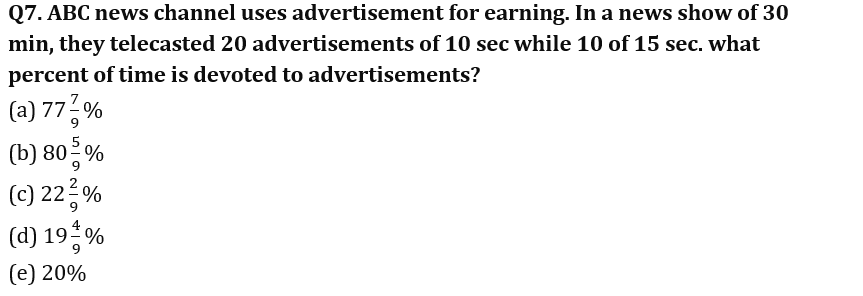Q8. The probability of solving a question by Jindal is 3/5 while that of not solving it by Avi ¼. What is the probability that the problem be solved?
(a) 3/5
(b) 7/10
(c) 1/5
(d) 3/10
(e) 3/20

Q9. Ritesh travels at 1 kmph to reach station 500m far from his house to catch a train. He started but after 4 minutes, he realized that he forgot a document at home so he returned with same speed. What should be his speed (in kmph) so that he catches the train?
(a)0.36
(b) 0.27
(c) 1.27
(d) 1.36
(e) 1.5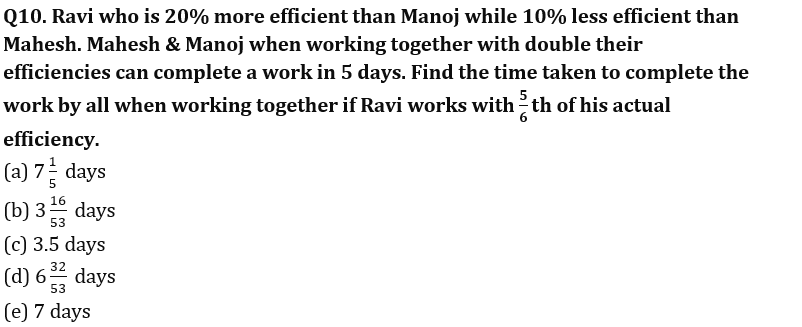Q11. In a bag, there are 16 balls of three different colors i.e. red, blue and green. Number of red and blue balls is 9 and difference between red and green ball is 4 then find the probability of getting a ball of each color if three balls are picked at random?
(a) 5/28
(b) 4/27
(c) 7/36
(d) 8/35
(e) 9/40

Q12. Cost price of article A is Rs 600 more than that of B and selling price of A is Rs 1200 more than that of B. If difference between profit earned on selling these two articles is 13⅓% of the cost price of A then find profit earned on B if profit % earned on A is 33⅓%?
(a) Rs 800
(b) Rs 900
(c) Rs 840
(d) Rs 960
(e) Rs 1000

Q13. In 100 lit mixture of milk and water, milk is 68 lit more than that of water. When ‘a’ lit of milk is taken out and (a+15) lit of water is added, milk becomes 50% more than that of water. Find value of ‘a’?
(a) 20
(b) 15
(c) 18
(d) 12
(e) 16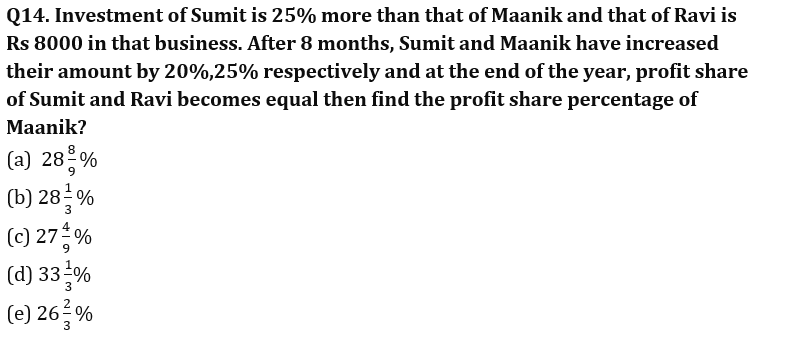Q15. Area of rectangle having length and breadth in the ratio of 4: 3 is 432 cm2. A square whose side is equal to diagonal of rectangle then find ratio of numerical value of perimeter to the area of square?
(a) 7:55
(b) 4:35
(c) None of these
(d) 1:8
(e) 2:15

Solutions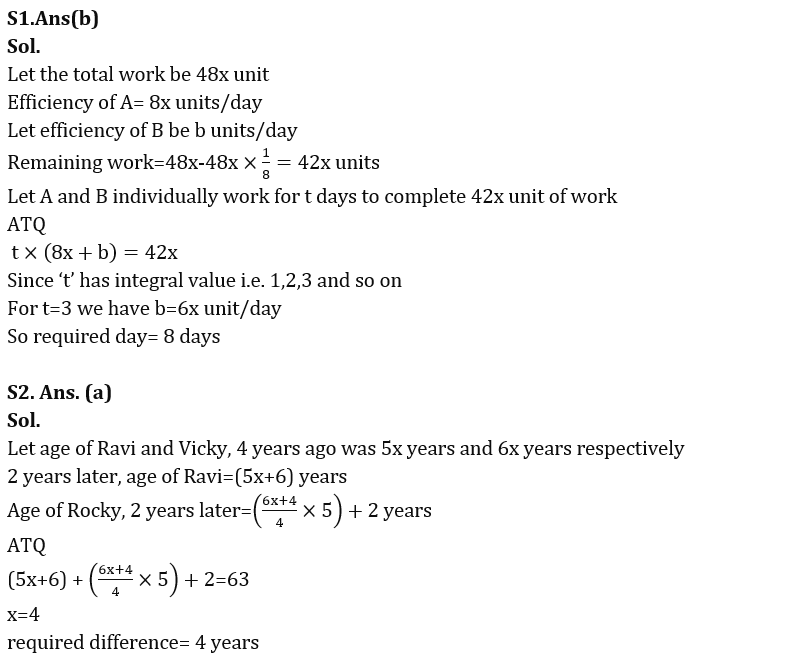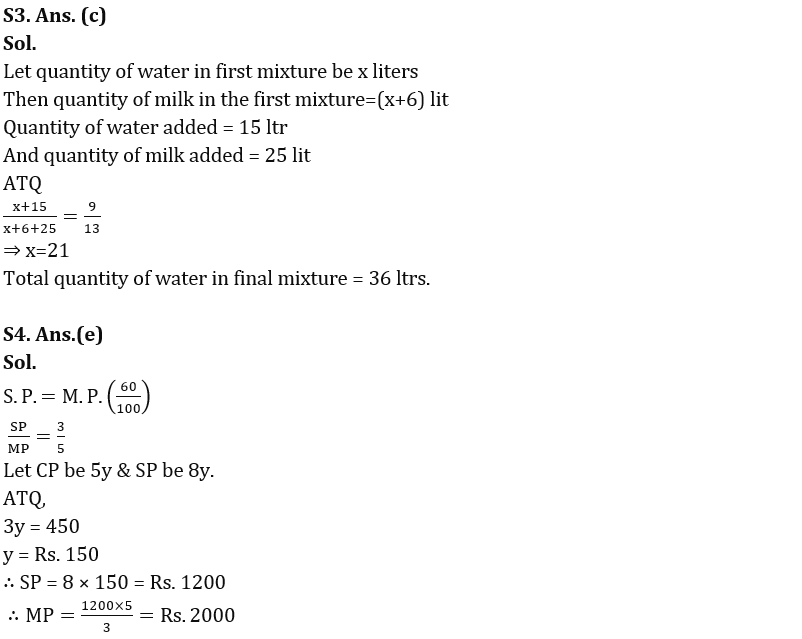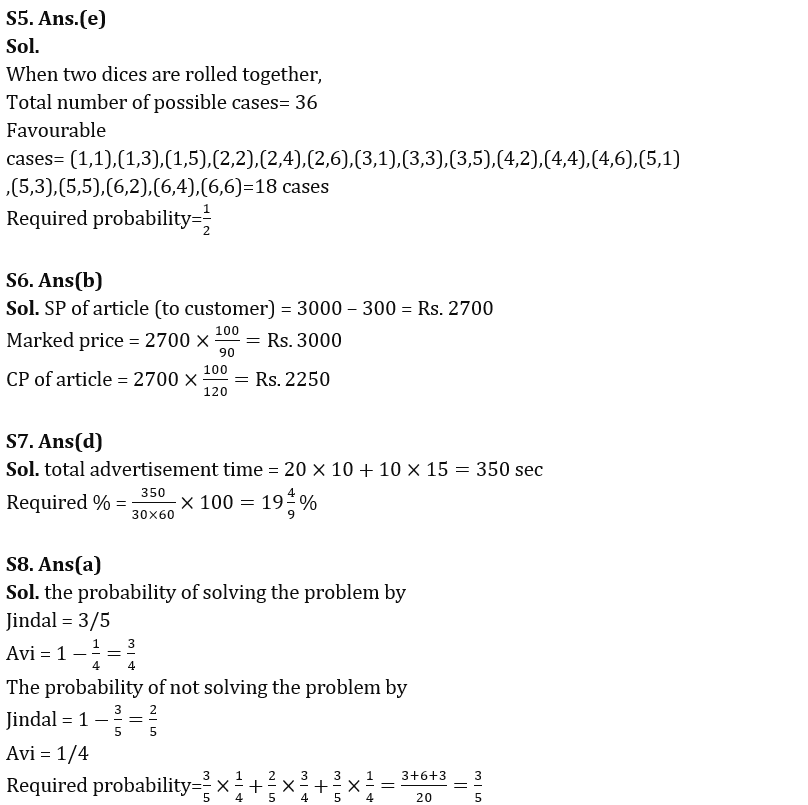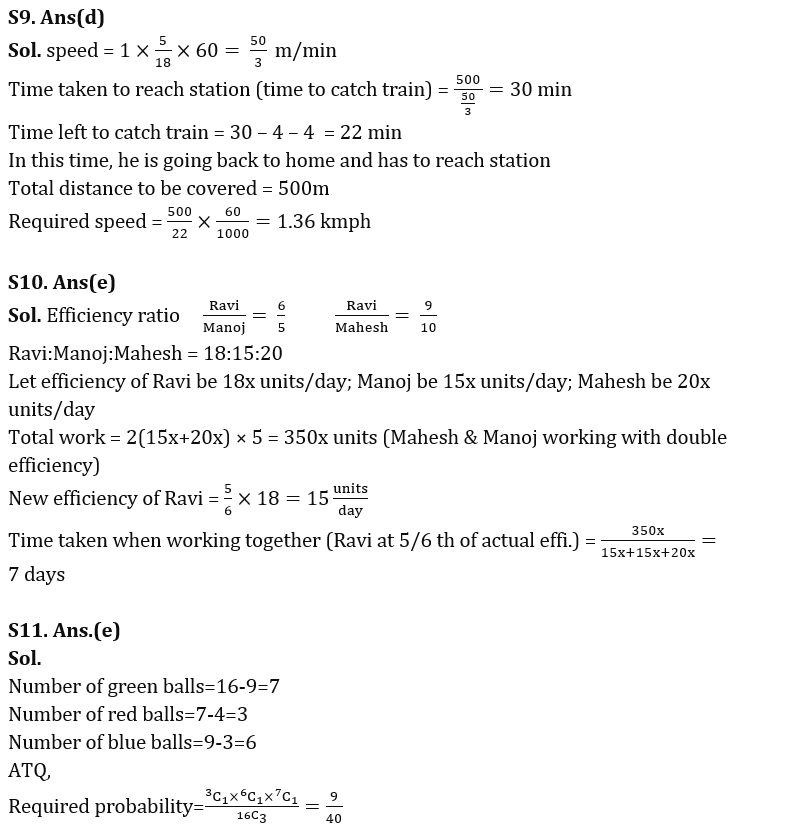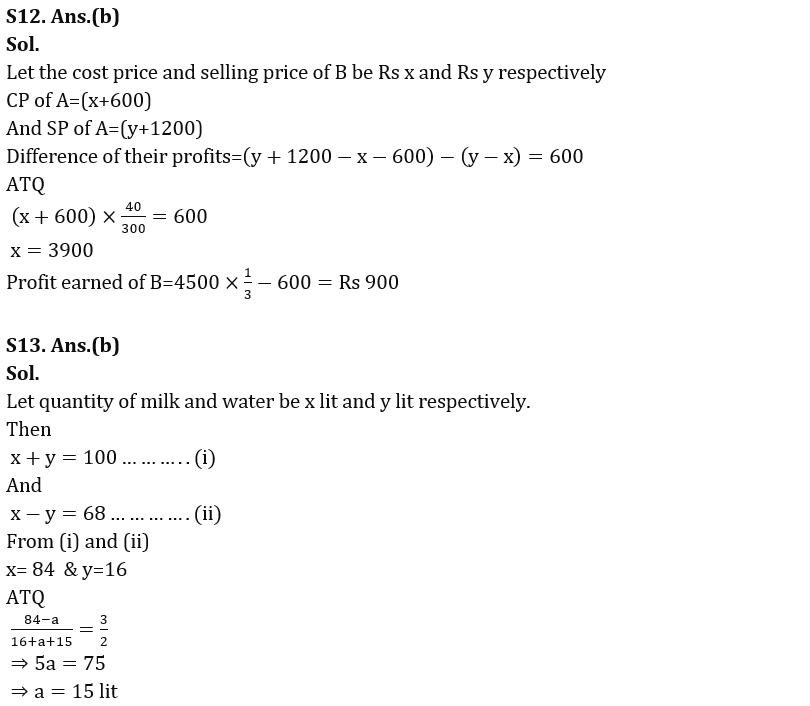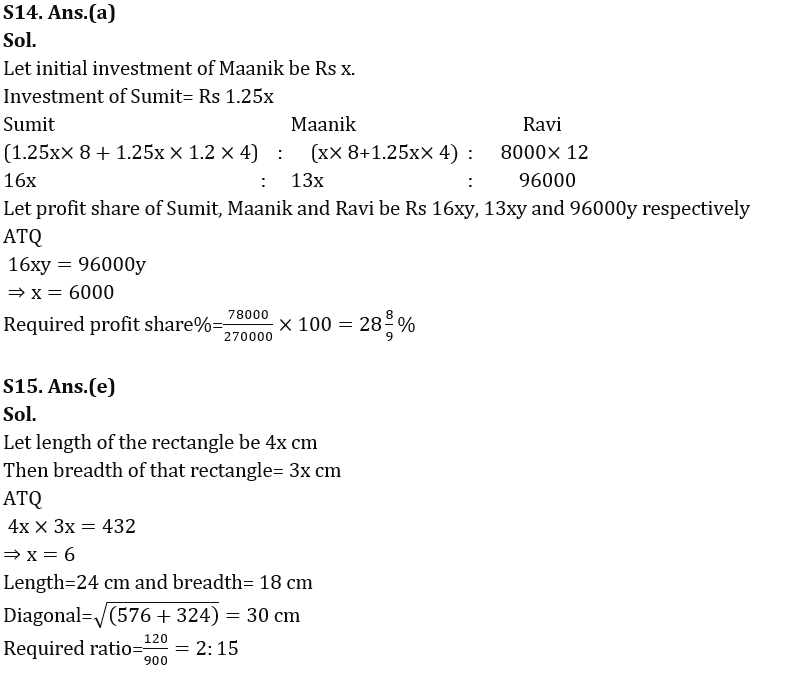## FAQs

#### Congratulations!